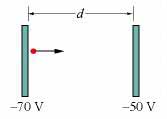# Charge particle between 2 paralleled plates

## Homework Statement

In the figure below, a charged particle (either an electron or a proton) is moving rightward between two parallel charged plates separated by distance d = 2.30 mm. The particle is speeding up from an initial speed of 3640 km/s at the left plate.## Homework Equations

The equation that I was given to use (The one my professor worked out on the board) is:

W(cons)=-ΔV=ΔK=-(-e)(ΔV)=1/2 m(particle)(Vf2-Vi2)

The first part of the problem was to find out if the particle was an electron or a proton. It is an electron.

## The Attempt at a Solution

1/2 (9.1*10-31)*36402= 6.02*10-24

This was not correct.

So... what's the question?

I need help in solving this problem. I cannot figure it out and the equation I was using did not work. What equation would you use to solve it?

gneill
Mentor
I need help in solving this problem. I cannot figure it out and the equation I was using did not work. What equation would you use to solve it?

Equation to find what? You haven't stated what it is you are trying to find. Where in your problem statement does it specify what it is that you looking for?

What is its speed just as it reaches the plate at the right?

gneill
Mentor
What is its speed just as it reaches the plate at the right?

So, in your solution attempt, what was the equation you used (it's general form; what does it calculate)?